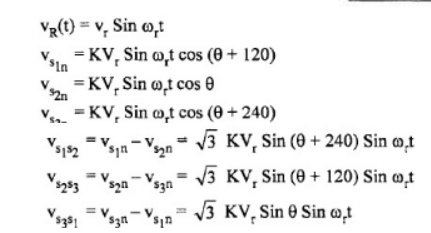# Synchros

A commonly used error detector of mechanical positions of rotating shafts in AC control systems is the Synchro.

Synchros

A commonly used error detector of mechanical positions of rotating shafts in AC control systems is the Synchro.

It consists of two electro mechanical devices.

o   Synchro transmitter

o   Synchro receiver or control transformer.

The principle of operation of these two devices is sarne but they differ slightly in their construction.

o   The construction of a Synchro transmitter is similar to a phase alternator.

o   The stator consists of a balanced three phase winding and is star connected.

o   The rotor  is  of  dumbbell  type construction and is  wound with  a  coil  to produce  a magnetic field.

o   When a no voltage is applied to the winding of the rotor, a magnetic field is produced.

o   The coils in the stator link with this sinusoidal distributed magnetic flux and voltages are induced in the three coils due to transformer action.

o   Than the three voltages are in time phase with each other and the rotor voltage.

o   The magnitudes of the voltages are proportional to the cosine of the angle between the rotor position and the respective coil axis.

o   The position of the rotor and the coils are shown in Fig.When q=900 the axis of the magnetic field coincides with the axis of coil S2 and maximum voltage is induced in it as seen.

For this position of the rotor, the voltage c, is zero, this position of the rotor is known as the 'Electrical Zero' of die transmitter and is taken as reference for specifying the rotor position.

In summary, it can be seen that the input to the transmitter is the angular position of the rotor and the set of three single phase voltages is the output.

The magnitudes of these voltages depend on the angular position of the rotor as givenNow consider these three voltages to he applied to the stator of a similar device called control transformer or synchro receiver.

The construction of a control transformer is similar to that of the transmitter except that the rotor is made cylindrical in shape whereas the rotor of transmitter is dumbbell in shape.

Since the rotor is cylindrical, the air gap is uniform and the reluctance of the magnetic path is constant.

This makes the output impedance of rotor to be a constant.

Usually the rotor winding of control transformer is connected teas amplifier which requires signal with constant impedance for better performance.

A synchro transmitter is usually required to supply several control transformers and hence the stator winding of control transformer is wound with higher impedance per phase.

Since the some currents flow through the stators of the synchro transmitter and receiver, the same pattern of flux distribution will be produced in the air gap of the control transformer.

The control transformer flux axis is in the same position as that of the synchro transmitter.

Thus the voltage induced in the rotor coil of control transformer is proportional to the cosine of the angle between the two rotors.

Study Material, Lecturing Notes, Assignment, Reference, Wiki description explanation, brief detail
Control Systems : Systems and their Representation : Synchros |